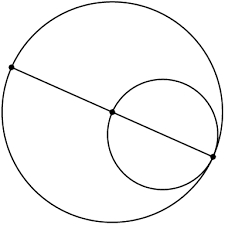# The ratio

The ratio of the lengths of the two circles is 5:2. Find the ratio of a
a) the radii of these circles
b) the areas for these circles

a =  5:2
b =  25:4

### Step-by-step explanation:Did you find an error or inaccuracy? Feel free to write us. Thank you!

Showing 1 comment:
Math student
Can we take pictures of the problem

Tips for related online calculators
Check out our ratio calculator.

#### Grade of the word problem:

We encourage you to watch this tutorial video on this math problem: